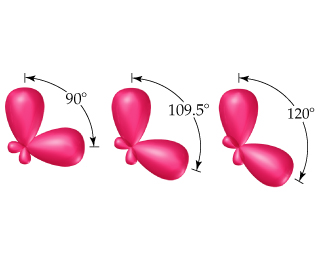# Problem: Shown here are three pairs of hybrid orbitals, with each set at a characteristic angle.  For each pair, determine the type of hybridization, if any, that could lead to hybrid orbitals at the specified angle.

###### FREE Expert Solution

We have to determine the type of hybridization for each of the given set of orbitals.

To determine the hybridization of the given orbitals, we will have a look at various hybridizations and their bond angles.

Various geometriesthat can result by arrangement of electron groups around a central atom are:

Electron Regions          Electronic Geometry          Hybridization            Bond Angles

89% (1 ratings)###### Problem Details

Shown here are three pairs of hybrid orbitals, with each set at a characteristic angle.For each pair, determine the type of hybridization, if any, that could lead to hybrid orbitals at the specified angle.